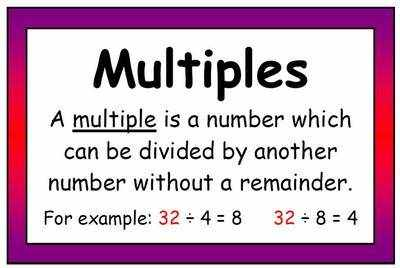Learn the 4 Things You Must Know Before Taking the GMAT!   Download it Now!

# GMAT Divisibility ProblemsCertain problem solving and data sufficiency questions on the GMAT concern what I call number theory, where you need to determine whether one number is a factor or multiple of another number. They can be tricky, but there’s a fundamental principle that, once you understand it, will help make these types of questions much easier for you.

Consider this example from Chili Hot GMAT (give it a try — you have 2 minutes…Go!):

Question: If P represents the product of the first 13 positive integers, which of the following must be true?

I. P is an odd number
II. P is a multiple of 17
III. P is a multiple of 24

(A) I only
(B) II only
(C) III only
(D) None of the above
(E) All of the above

First things first, hopefully you used your scratch paper to visualize what P is:

P = 1 x 2 x 3 x 4 x 5 x 6 x 7 x 8 x 9 x 10 x 11 x 12 x 13
Note: This is also equivalent to 13! (or 13 factorial)

Evaluating Statement 1 first, you should recognize it as false because as long as there is at least one even number in a multiplication sequence, the entire product will be even.

Remember these rules:

Even x Even = Even
Even x Odd = Even
Odd x Odd = Odd

Once you determine that Statement 1 is false, of course, you can eliminate both answer choices A and E.

Now, Statements 2 and 3 are considerably more difficult. Well, maybe. Actually, it becomes easy to determine whether 17 or 24 (or any number, for that matter) is a factor of P once you learn this fundamental principle of divisibility:

Rule: Any number x will not be a multiple of y if y contains any distinct prime number not included in x.

The corollary to this is: x will be a multiple of y only if x contains, at a minimum, the same number of distinct prime factors as y does.

For example, the number 6 is a multiple of 3 because 3 contains no prime numbers that aren’t already included in 6 (i.e. 3). The number 6 is not a multiple of 5 because 5 has a prime factor, 5, which is not shared with the prime factors of the number 6.

Now back to our problem. Let’s look at Statement 2. Based on the divisibility principle above, is 17 a factor of P?

Well, the distinct prime factor of 17 is, simply, 17 (because the prime factorization of 17 is 17 = 1 x 17).

The prime factors of P include 2, 3, 5, 7, 11, and 13.

Does the number 17 therefore contain any distinct prime factors not included in P? Of course. The prime number 17 is included in 17 but not in P. Therefore, P is not a multiple of 17. Statement 2 is false.

Applying the same logic to Statement 3, we already know that the prime factors of P are 2, 3, 5, 7, 11, and 13.

What are the distinct prime factors of 24? Well the prime factorization of 24 = 1 x 2 x 2 x 2 x 3. Thus, the distinct prime factors of 24 are 2 and 3.

Are there any prime factors in 24 that aren’t in P? No. In other words, P contains all of the distinct prime numbers that 24 has, and therefore P is a multiple of 24. Statement 3 is true.

The answer to the question, therefore, is C.

This problem helps reveal the mathematical reason for why one number is or is not a multiple of another number. Make sure you understand the divisibility rules explained above, as they’ll help you to answer numerous questions of this type on the GMAT!